Exact differential equations

Exact Differential Equations

Review on partial derivatives

Before we start on the topic of exact differential equations, it is important for you to know how to work with partial derivatives.
At this point you should be well acquainted with the process of total differentiation, in other words, you know how to take total derivatives of a function in terms of a certain variable, with respect to that variable. But depending on our level of studies and the topics we are working on, you will encounter functions which are defined in terms of more than one variable, and even sometimes, one of these variables is a function in terms of the other. In these cases differentiation will require you to work through the derivatives of the different variables in different ways depending on the variable you are differentiating with respect of.

For such functions defined in terms of multiple variables, you can also take partial derivatives. Partial differentiation means that you will select one of the variables the function is defined in, and differentiate only respect to this particular value by taking any other variable involved as if it was a constant. You may be already familiarized with this process (or maybe even know how to solve these operations), just in case, we will have a quick review for this section, so the formulas and the process of exact equations is well established later.

The main thing to remember is that total differentiation acts on all the variables inside a function, while partial differentiation acts only on the one that is being differentiated with respect of, and leaves the rest as constants while operating. Note, this does not mean that the variables treated as constants through partial derivation are converted into constants for the remainder of the problem, they continue to be variables and used as such, is just during the partial differentiation process that we make this distinction.

To see the distinction between total and partial differentiation clearly let us work through a function we will call psi, which is in terms of x and y as follows: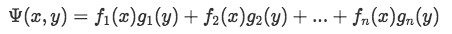Equation 1: Psi function in terms of 2 variables (x and y)

If we are to take the derivative of psi with respect to x our result would be: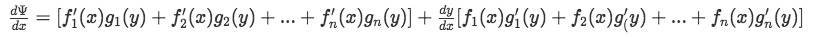Equation 2: Total derivative of Psi respect to x

Which can be rewritten as: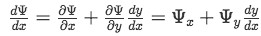Equation 3: General formula for the total differentiation of Psi respect to x

Notice that when we say "take the derivative" we mean the total derivative. Unless explicitly mentioned as taking the "partial" derivative (for cases that require it so), we usually follow the total differentiation process.

From equations 2 and 3 we can already see what partial differentiation is all about since: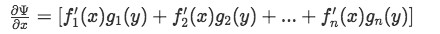Equation 4: Partial derivative of Psi respect to x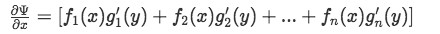Equation 5: Partial derivative of Psi respect to y

When partially deriving a function, we take as constants the variables we are not deriving with respect of, just like we took as a constant the function in terms of y in equation 4, and the function in terms of x in equation 5, while for the total differentiation of Psi (equation 2) we derived each term on the function's expression by the variable in question. In this case you can see how the function g(y) is also derived with respect of x even when g is in terms of y. This is done because y in itself can very well be either a constant or a function in terms of x, whatever it is, we need to derive it and find out the result.

For practical purposes, partial derivatives can be written as: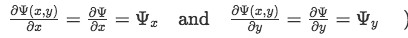Equation 6: Different notation forms for partial derivatives

To finish this section, now you know that depending on the information that we are looking for from a function, we can operate on it in different ways. When talking about derivation, remember you can have total derivatives and partial derivatives. They are not the same and they have different notation, make sure you understand and remember these differences since a single mistake in notation can change the complete meaning of the operation you are applying to the function.

We will continue to use both types of differentiation on this topic, make sure you always understand which operation is being applying each time.

What is an exact equation?

We define an exact differential equation as that which its final solution is a continuous function equal to a constant. This happens to be a very simple concept that you are already familiarized with. Simply said, you already know that whenever you take the derivative of a constant, the result will be zero.

Well, we will now use this basic knowledge to solve a differential equation that may seem much more complicated. In general, when we solve an exact differential equation, this will start in the form of: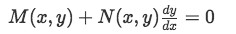Equation 7: General form of an Exact Differential Equation

If you notice, this is highly resemblant to equation 3:Equation 3: General formula for the total differentiation of Psi respect to x

So we can actually relate these two equations by setting the total derivative of Psi with respect to x equal to equation 7: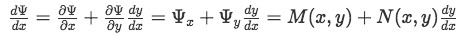Equation 8: Setting the total derivative of Psi equal to the general form of an Exact Differential Equation

And so, this would mean we can relate M and N to the partial derivatives of Psi with respect to x and y each: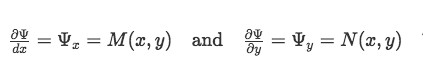Equation 9: Setting the partial derivatives of Psi equal to M and N

So the final expression we would need to solve is: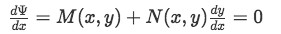Equation 10: General form of an exact differential equation related to the total differentiation of Psi

From which we can automatically conclude that the continuous function Psi is a constant since its total differentiation is equal to zero: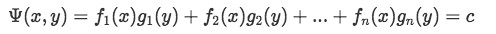Equation 11: Function Psi is equal to a constant

To summarize, we define exact those equations with a solution equal to a continuously differentiable function which happens to have a total differential equal to zero. For the case of Psi:

Which in turn results as a zero for either x or y total differentiations of Psi (we can see the x total differentiation in equation 10), and thus, defines the function Psi as a constant. To put it in simpler words, whenever you find the solution to exact equations, the solution will be equal to a constant.

How to solve exact differential equations

Now that we have seen what exact differential equations are, is time for us to learn how to find the general solution of the differential equation in this form.

The first step to solving a differential equation that seems to be exact, is to check if it is indeed an exact equation. If it turns out to be a different type of differential equation we cannot use this method to find its solution, and therefore we need to go back and check for other techniques such as the separable equations method.

The condition for a differential equation to be exact comes from the partial derivatives of M and N (or in other words, the mixed partial derivatives of Psi). The reasoning behind it comes from the characteristics of the function we are trying to solve for, so in this case, it comes from Psi being continuously differentiable. When a function is continuously differentiable, it is said that if you differentiate it twice, once for each of its variables, it doesn't matter the order in which you differentiate, the result will be the same. Mathematically speaking: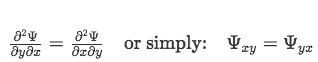Equation 13: Property of mixed partial derivatives operating on a continuous function

Notice that :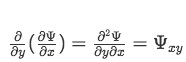Equation 14: Mixed partial derivatives notation guidelines

For our general form of an exact equation (go back and check equation 9), this translates into:

And so the condition for equation 10 to be an exact differential equation is that: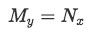Equation 16: Condition for an exact differential equation

Still, there will be occasions in which an equation will not be exact, in such cases we could use an integrating factor to convert it into one, this is a process we call: "Integrating factor for exact equations". We will look at the integrating factor method in the next StudyPug section, for now we will focus on functions which are already exact.

So how to solve exact equations? Let us look into a first example where we can observe the process step by step.

Example 1

Having the initial condition of y(0)=2 for the equation: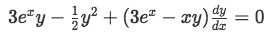Example 1: Solve the differential equation

First let us check if this equation is exact and at the same time practice how to do partial derivatives: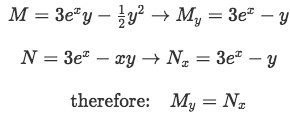Example 1(a): Proof that the differential equation is exact

Notice that in the partial derivative of M with respect to y, we took x as a constant and only differentiated by y. In the same way, in the partial derivative to N with respect of x, we took y as a constant and only differentiated by x.

Since the equation is an exact equation, now we can continue to solve it.
In order for us to find the function Psi, which is the solution to the differential equation, we take either M or N and integrate it. How does this work? If you remember, M is the partial derivative of Psi with respect to x and N is the partial derivative of Psi with respect to y, therefore we take the approach of reverse engineering and integrate the differentiations to get back the original function.

This is simpler said than done, because if we pick M and integrate it, we have to integrate with respect of x, in the other hand, if we pick N and integrate it, we have to integrate with respect of y. The math itself is easy, but it can be tricky and confusing, therefore we recommend caution while going through the process.

In order for you to see how it doesn't matter if you pick either M or N to integrate it and find Psi we will work through both of them in this article.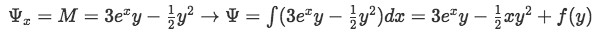Example 1(b-1): Finding Psi by integrating M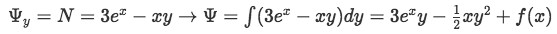Example 1(b-2): Finding Psi by integrating N

Let us continue first with the path on Example 1(b-1):
Observe you have a function of y at the end of the right hand side on Psi. The reason for it is that if you were to take the partial derivative of this term with respect to x (to make M) it would be treated as a constant and you would obtain a zero. Now we partially derive this Psi with respect of y: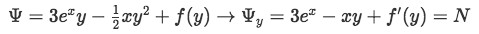Example 1(c-1): Finding f(y) by partially deriving Psi with respect to y

So we know that f'(y)=0, therefore f(y) MUST be a constant.

We follow the exact same steps but now for the equation in Example 1(b-2). Just like before, the right hand side contains a function of x that would have been treated as a constant when you derive to obtain N. So we partially derive to obtain: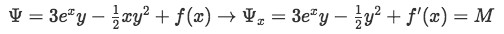Example 1(c-2): Finding f(x) by partially deriving Psi with respect to x

So f'(x) is equal to zero and therefore f(x) MUST be a constant too.

And thus, equations of Example 1 (b-1) and (b-2) become: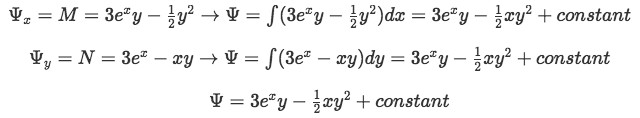Example 1 (d): Finding Psi by integrating its known partial derivatives

As you can see, either you pick M or N makes no difference, the result of the integral is the same function, which is Psi! Something important to note is that you have a constant in the right hand side that you can just dismiss since it will make no proportional difference to the result, and thus, for practical purposes we just took it away. In some other cases the functions at the end of the integration result may not be constants, so be careful to not miss those.

Now the last step to solve the differential equation is to find the value of the function. Remember that Psi is in fact a constant c and so, we will use the initial condition of y(0)=2 to find its value.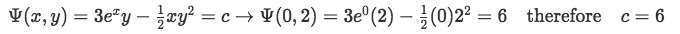Example 1(e): Finding the value of Psi using the initial condition given

And we are done! We have found the solution of the differential equation for example 1.

So let us summarize the steps of the technique of exact equations:

1. Identify M and N in the differential equation and check if the given equation is exact.
2. If exact, pick M or N and integrate it to find Psi.
1. If you pick M, integrate with respect to x. The result will contain a last term which will be a function in terms of y.
2. If you pick N, integrate with respect to y. The result will contain a last term which will be a function in terms of x.
3. Although we did it for clarification purposes, you don't have to work through both parts a and b of this step. Doing either one or the other is enough.
3. Once you have gotten your preliminary result of Psi following the step above, partially derivate your Psi in order to know more about the function at the end.
1. If you followed step 2.a then you will have to partially derivative Psi with respect to y. The resulting expression will be equal to N and you will be able to infer if f(y) was a constant or not.
2. If you followed step 2.b then you will have to partially derivative Psi with respect to x. The resulting expression will be equal to M and you will be able to infer if f(x) was a constant or not.
3. Remember, if you followed step 2.a you only need to follow step 3.a. In the same way if you followed step 2.b you only follow step 3.b.
4. Having found the unknown function at the end, set it up in your Psi and then apply the initial conditions to find the final value of Psi.

If the process seems confusing to you, do not worry, with practice you will be able to handle such problems easily. In the next section of this article we present a few more examples for you to work through and develop your skills. For more on this topic we recommend you to visit these differential equations notes or these examples on exact differential equations.

Solving exact differential equations

To end this article we will tackle a second and third differential equations examples with the method of exact equations. For example 2 we will follow steps 1, 2.a, 3.a and 4, and for example 3 we will follow steps 1, 2.b, 3.b and 4, so you can observe how you can follow either one path or the other and obtain the final result.

Example 2

• Let's start with equation below with initial conditions of y=1, x=1.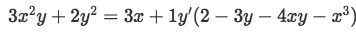Example 2: Solve the differential equation
• Step 1:

We rearrange the differential equation in order to identify M and N and then check if is exact.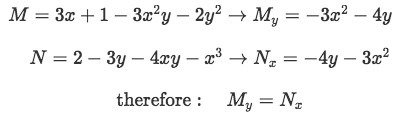Example 2(a): Proof that the differential equation is exact
• Step 2:

We pick M to integrate and find Psi: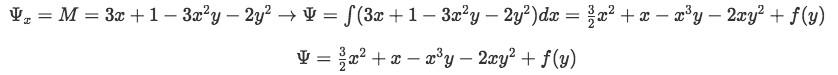Example 2(b): Finding Psi by integrating M
• Step 3:

Obtain the partial derivative of Psi with respect to y to find f(y):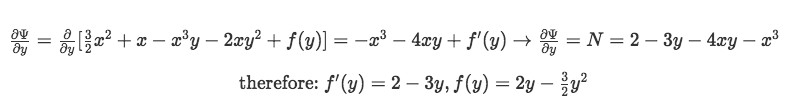Example 2(c): Finding f(y) by partially derivating Psi with respect to y
• Step 4:

Having found f(y), we can write the final equation for Psi: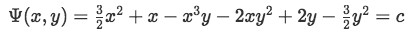Example 2(d): Final equation for Psi

We apply the initial conditions y=1 and x=1 to find the final value of Psi: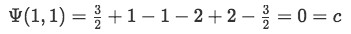Example 2(e): Finding the value of Psi with the initial conditions

And so the final solution to the differential equation in example 2 is: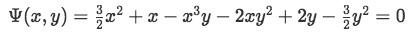Example 2(f): Final solution of the differential equation

Example 3

• Having the equation below with the initial condition of y(0)=2Example 3: Solve the differential equation
• Step 1:

Identify M and N, and check if the equation is exact: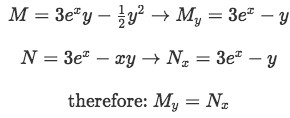Example 3(a): Proof that the differential equation is exact
• Step 2:

We pick N to integrate and find Psi: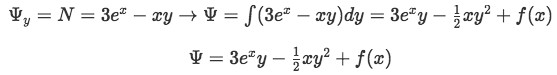• Step 3:

Obtain the partial derivative of Psi with respect to x to find f(x):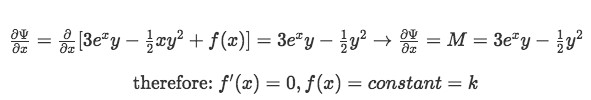Example 3(c): Finding f(x) by partially derivating Psi with respect to x
• Step 4:

We rewrite the final Psi and apply the initial condition:

Notice that for the first line of the equation for example 3(d) we have written k in both sides of the first equal sign. The reason we have done this is that the full equation of Psi contains that constant k, and it will give as a total result another constant (which we have called C) since is an exact equation. We can actually dismiss the k constant since it will have no effect on the rest of the equation thanks to proportionality, which means that if we dismiss k from the left hand side of the equation, we have to dismiss it too from the right hand side, and that would mean to subtract k from C.

Notice that we don't know what C is, is just a constant too, so the result from C-k would be another constant we will call c. Since all of these are constants, we could very well just have dismissed k from the beginning and left Psi equal to c directly (this is what we did on example 1) but we wanted to explain this process explicitly so you could observe that since all k, C and c are unknown constants, we can manage them easily.

• Now let us apply the initial condition y(0)=2 to find c: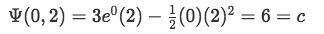Example 3(e):Finding the value of Psi with the initial conditions

So our final solution is: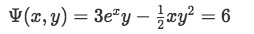Example 3(f): Final solution of the differential equation

If you are interested in seeing a different approach to the method of exact differential equations, we propose you visit this backwards" approach to exact equations", where you will see how others have solved these same type of problems using a kind of reverse engineering technique under the same principles we have described here.

Exact differential equations

Lessons

Recall Partial Derivatives:

Notation:

$\frac{dF}{dx}=F_x$ or
$\frac{dF}{dx}=F_y$

Exact Differential Equations:
Sometimes a differential equation is not solvable via the separable equations technique.

Let's suppose the differential equation we want to solve is of the form:

$M(x,y)+N(x,y) \frac{dy}{dx}=0$

Now let's imagine we have another function of $x$ and $y$, denoted as $\Psi (x,y)$ "psi".

It is demonstrated throughout the video that: $\frac{d}{dx} \Psi(x,y)=\frac{\partial\Psi}{\partial x}+\frac{\partial\Psi}{\partial y}\frac{dy}{dx}$

So if we can find a $\Psi(x,y)$ such that $\Psi_x=M(x,y)$ and $\Psi_y=N(x,y)$, then

$\frac{d}{dx} \Psi(x,y)=\frac{\partial\Psi}{\partial x}+\frac{\partial\Psi}{\partial y}\frac{dy}{dx}=$ $\Psi_x+\Psi_y \frac{dy}{dx}=$$M(x,y)+N(x,y) \frac{dy}{dx}=0$

Given that $\frac{\partial \Psi}{\partial x} \neq \frac{d \Psi}{dx}$

So our solution to solving the differential equation is just

$\Psi(x, y)=c$

Finding this $\Psi(x, y)$:

Ok, so provided that $\Psi(x, y)$ is continuous and it's first derivative is continuous as well, we should have the following equality hold

$\Psi_{xy}=\Psi_{yx}$

(Remember this from Calculus 3, if not just trust me!)

So,

$\Psi_{xy}= (\Psi_x)_y=(M)_y=M_y$
$\Psi_{yx}= (\Psi_y)_x=(N)_x=N_x$
$\Longrightarrow M_y=N_x$

So a differential equation will be exact if and only if $M_y=N_x$

Then we can solve for our $\Psi(x, y)$ by integrating $M$ with respect to $x$, or $N$ with respect to $y$.

And once we have $\Psi(x, y)$ we have solved the differential equation just equate:
$\Psi(x, y)=c$ and that will be the answer
• Introduction
a)
Partial Differentiation Review

b)
What are Exact Differential Equations and how do we solve them?

• 1.
Partial Differentiation
Find the first order partial derivative of every variable for the following functions:
a)
$f(x,y)=3x^2-3y+2$

b)
$N(y,z)=2y^2 z$

• 2.
Determining what is an Exact Differential Equation
Solve the following differential equation

$2xy^2-y^2+(2x^2 y-2xy) \frac{dy}{dx}=0$

Using the equation: $\Psi(x,y)=x^2 y^2-xy^2=2$

• 3.
Solving Exact Equations
Solve the following differential equation

$3e^x y- \frac{1}{2} y^2+(3e^x-xy) \frac{dy}{dx}=0$

With initial conditions $y(0)=2$

• 4.
a)
Solve the following differential equation

$3x^2 y+2y^2=3x+1+y' (2-3y-4xy-x^3)$

With initial conditions $y=1, x=1$

b)
Verify that the solution you found is in fact the solution to the above differential equation (may be useful to do on a test).